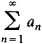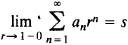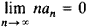# Tauberian Theorem

The following article is from The Great Soviet Encyclopedia (1979). It might be outdated or ideologically biased.

## Tauberian Theorem

If an infinite series is summable or an integral is integrable by a certain method, it is of interest to know under what conditions summability or integrability may be obtained for a weaker method (see). The conditions that must be imposed on the series or integral are established by theorems known as Tauberian theorems.

One of the first such theorems was proved by A. Tauber in 1897. It states that if for the series of numbersthere exists the limit(that is, if the series is summable to s by the Abel method) and ifthen the series converges to s.

Tauberian theorems are used in many fields of mathematics, particularly in analytic number theory and the study of the asymptotic behavior of the eigenvalues and eigenfunctions of differential operators.